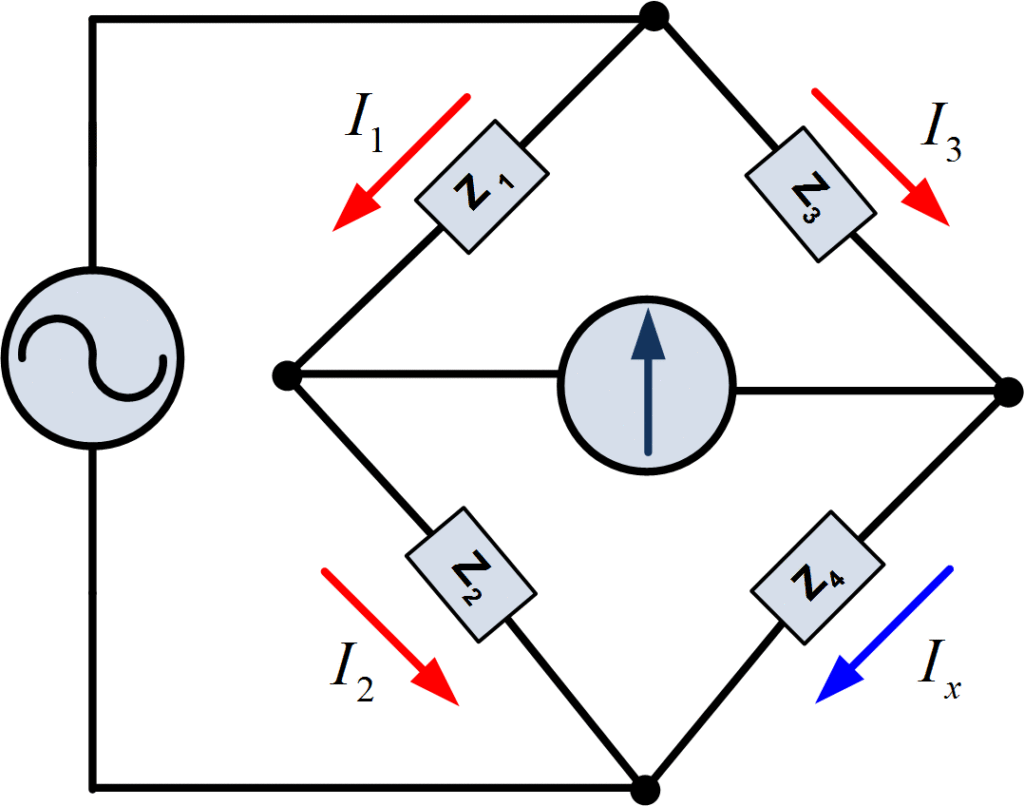Home / Instrumentation / Impedance Measurement Theory

# Impedance Measurement Theory

Want create site? Find Free WordPress Themes and plugins.

The opposition that a circuit offers to the flow of AC is called impedance. By measuring the voltage and current in an AC circuit and utilizing the following equation

$Z={}^{V}/{}_{I}$

We can obtain the magnitude of circuit impedance. However, it is often desirable to separate impedance into resistive and reactive components. One instrument that is used to measure the separate resistive and reactive parts of an impedance is the AC Bridge. The circuit of the general AC Bridge is shown in figure 1.Fig.1: The AC Bridge Circuit

The configuration is similar to that of the Wheatstone bridge, yet distinct differences exist between the components. The AC Bridge has impedance arms, rather than resistance arms; instead of a battery and galvanometer, an AC signal source and null detector are used. If the signal voltage is in the audio range, a set of headphones may be used as the null detector; otherwise, a sensitive AC Voltmeter is used.

As in the Wheatstone bridge, at balance, no current flows through the detector. The voltage from a to c equals that from a to d, so that

${{I}_{1}}{{Z}_{1}}={{I}_{3}}{{Z}_{3}}\text{ (1)}$

Similarly,

${{I}_{2}}{{Z}_{2}}={{I}_{x}}{{Z}_{x}}\text{ (2)}$

It follows that for balanced conditions

$\frac{{{Z}_{1}}}{{{Z}_{2}}}=\frac{{{Z}_{3}}}{{{Z}_{x}}}$

${{Z}_{x}}={{Z}_{3}}\left( \frac{{{Z}_{2}}}{{{Z}_{1}}} \right)\text{ (3)}$

In order to obtain balance, at least one of the unknown impedances must have a resistive and reactive components. If Z3 is chosen to be complex, Z1 and Z2 can be conveniently chosen to be purely resistive with a ratio that is a power of 10. Then from equation (3),

${{R}_{x}}+j{{X}_{x}}=({{R}_{3}}+j{{X}_{3}})\left( \frac{{{R}_{2}}}{{{R}_{1}}} \right)\text{ (4)}$

For equation (4) to be satisfied, the real parts of both sides must be equal, and also the imaginary parts of both sides. Equating these parts, we obtain

${{R}_{x}}={{R}_{3}}\left( \frac{{{R}_{2}}}{{{R}_{1}}} \right)\text{ (5)}$

${{X}_{x}}={{X}_{3}}\left( \frac{{{R}_{2}}}{{{R}_{1}}} \right)\text{ (6)}$

A simple inductance bridge is shown in figure 2.Fig.2: An Inductance Bridge

When the bridge is balanced, we solve for Rx by using equation (5). From equation (6), we then solve for the inductance Lx,

$\omega {{L}_{x}}=\omega {{L}_{3}}\left( \frac{{{R}_{2}}}{{{R}_{1}}} \right)\text{ }$

Or

${{L}_{x}}={{L}_{3}}\left( \frac{{{R}_{2}}}{{{R}_{1}}} \right)\text{ (7)}$

Here, from equation (7), we can easily obtain the unknown inductance in the circuit.

Did you find apk for android? You can find new Free Android Games and apps.

### About Ahmad FaizanMr. Ahmed Faizan Sheikh, M.Sc. (USA), Research Fellow (USA), a member of IEEE & CIGRE, is a Fulbright Alumnus and earned his Master’s Degree in Electrical and Power Engineering from Kansas State University, USA.